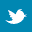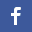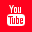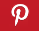Completely Solved C, C++ Programs Assignment.Quick Search Database Videos Tutorials Ebooks Forums FAQ Aboutus Household Industrial Manufacturing Service Shopping Transportation### Assessment System [SOLVED]:C++ Program

 Filed Under: C++ Assignments,Miscellaneous,Sample Assignments

Problem Statement: Assessment system
You are required to write a program for Assessment system of a University. The basic idea is to calculate the GPA (Grade Point Average) of each subject and then calculate the GPA of whole semester and on the basis of that GPA calculate the grade and remarks.
Detailed Description:
You are required to take the student’s marks for three subjects(English, Calculus, and Computer) as input from the user. After that calculate the GPA of each subject and the whole semester. Assume that total marks of a subject are 100 and each subject is of 3 credit hours.
Complete Assessment system is given below:
A 4.00 100 % Excellent
B 3.00-3.99 75-99 % Good
C 2.00-2.99 50-75 % Satisfactory
D 1.6-1.99 40-49 % Pass
F 0.00 Below 40 % Fail
• GPA for a subject = (obtained marks / 100) * 4 where 100 is the total marks of a subject.
NOTE: If user enters less than 40 marks for a subject, the GPA of that subject should be 0.
• GPA of whole semester = multiply each grade point you receive by the number of credit hours for that course, add up the totals, and then divide by the total number of credit hours taken in that semester.
• On the basis of Semester GPA, you have to give grade and remarks to the student
• If a student has less than 40 marks in a subject, a message should display that “you have to repeat this subject “.
• After displaying the GPA, grade and remarks, the program should ask the user if he/she wants to perform this task again. The user will be given two options (yes / no). If user enters “Y or y”, the program will start calculating the GPA, grade and remarks again. If user selects “N or n”, the program will terminate.
• If user enters Y or y as choice, the screen must be cleared before starting the whole process again. For this purpose you can use system(“cls”) function by including header file in the program.
Program Listing:
#include<iostream.h>
#include<conio.h>
#include<string.h>
int main()
{
char remarks;
int cal_marks=0,comp_marks=0,eng_marks=0;
float cal_gpa=0,comp_gpa=0,eng_gpa=0,total_gpa=0;
do{
cout<<”*********Assesment system of a University***********”;
cout<<”nEnter marks of computer:”;
cin>>comp_marks;
cout<<”nEnter marks of calculas:”;
cin>>cal_marks;
cout<<”nEnter marks of english:”;
cin>>eng_marks;
if(cal_marks<40)
{
cal_gpa=0;
}
else
{
cal_gpa=(cal_marks*4)/100;
}
if(comp_marks<40)
{
comp_gpa=0;
}
else
{
comp_gpa=(cal_marks*4)/100;
}
if(eng_marks<40)
{
eng_gpa=0;
}
else
{
eng_gpa=(cal_marks*4)/100;
}
total_gpa=(3*(cal_gpa+comp_gpa+eng_gpa))/9;
if(total_gpa==4)
{
char a=”Excellent”;
strcpy(remarks,a);
}
else if(total_gpa>=3)
{
char a=”Good”;
strcpy(remarks,a);
}
else if(total_gpa>=2)
{
char a=”Satisfactory”;
strcpy(remarks,a);
}
else if(total_gpa>=1.6)
{
char a=”Pass”;
strcpy(remarks,a);
}
else{
char a=”Fail”;
strcpy(remarks,a);
}
if(cal_gpa==0)
{
cout<<”nYou have to repeat calculas”;
}
if(comp_gpa==0)
{
cout<<”nYou have to repeat computer”;
}
if(eng_gpa==0)
{
cout<<”nYou have to repeat English”;
}
cout<<”nDo you want to calculate another result?(y/n):”;
cin>>a;
system(“cls”);
}while(a==’y'||a==’Y');
}